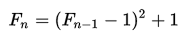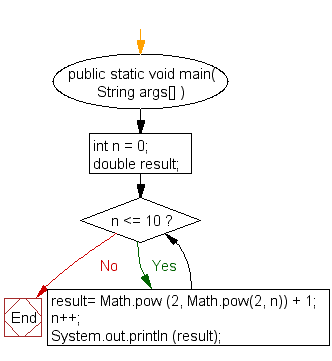﻿ Java exercises: Display first 10 Fermat numbers - w3resource# Java Exercises: Display first 10 Fermat numbers

## Java Numbers: Exercise-20 with Solution

Write a Java program to display first 10 Fermat numbers.

In mathematics, a Fermat number is a positive integer of the formwhere n is a nonnegative integer.
The first few Fermat numbers are:
3, 5, 17, 257, 65537, 4294967297, 18446744073709551617, …

Pictorial Presentation:Sample Solution:

Java Code:

``````import java.util.Scanner;
public class Example20 {

public static void main( String args[] ){
int n = 0;
double result;

while (n <= 10) {
result= Math.pow (2, Math.pow(2, n)) + 1;
n++;
System.out.println (result);
}
}
}
```
```

Sample Output:

```3.0
5.0
17.0
257.0
65537.0
4.294967297E9
1.8446744073709552E19
3.4028236692093846E38
1.157920892373162E77
1.3407807929942597E154
Infinity
```

Flowchart:Java Code Editor:

Contribute your code and comments through Disqus.

What is the difficulty level of this exercise?

﻿